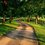# [Number Theory] Using Brahmagupta Identity and FTA to show Non-Negative Univariate Polynomial is SOS

Let's use the Fundamental Theorem Algebra (FTA) and the Brahmagupta Fibonacci identity to prove that every non-negative polynnomial $f(x) \in \mathbb{R}\left[ x \right]$ may be written as a sum of squares of polynomials.

Let $p \in \mathbb{R}[x] ~ \forall x \in \mathbb{R}$ and $p(x) \geq 0$. By the Fundamental Theorem of Algebra, a degree $n$ polynomial $p$ has exactly $n$ complex roots, and our polynomial $p$ is of the form constant times a product of positive quadratics. The graph of the polynomial lies entirely above the x-axis, or touches it. So in the first case, the roots of p are all complex, whereas in the latter, $p$ has real roots with even multiplicity. Hence our polynomial is of the form

$p = a~f_1^2f_2^2 \cdots f_n^2g_1 \cdots g_m,$

where $a$ is a real coefficient, the $f_i$'s have degree 1, and $g_j$'s have degree 2 and no real roots. We also note that by the complex conjugate root theorem, the roots of $p$ come in complex conjugate pairs. Also, $p(x)$ does not intersect the x-axis, so the degree of $p$ must be even.

A monic positive quadratic $g_j$, with no real roots, can be written as $(x+a)^2+b^2$ by completing the square. From here, an expression of $p$ as a sum of squares follows from the Brahmagupta-Fibonacci identity; the product of $g_j$'s, hence a product of the sums of squares, can be written as the sum of squares. For example, the product of two quadratics

$((x+a)^2+b^2)((x+c)^2+d^2)$

$= ((x+a)(x+c) + b^2d^2) + ((x+a)^2d^2-b^2(x+c)).$

If the degree of $p$ is 2, then

$p = (x-(a+ib))(x-(a-ib))$

$= x^2-2ax+a^2+b^2 = (x-a)^2+b^2$

where $a,b \in R[x],$ hence the sum of two squares.

If degree of $p$ is greater than or equal to 4, then we can write $p$ as the product of quadratics. By applying the Brahmagupta-Fibonacci identity as needed, the product of many sums of squares can be written as a sum of many squares.

Since the degree of $p$ is not odd, we have shown that a non-negative univariate polynomial is a sum of squares of polynomials. $\blacksquare$

Note that in the case that $p=a$, where $a\in \mathbb{R}$, $p$ is itself a square.Note by Bright Glow
3 years, 2 months ago

This discussion board is a place to discuss our Daily Challenges and the math and science related to those challenges. Explanations are more than just a solution — they should explain the steps and thinking strategies that you used to obtain the solution. Comments should further the discussion of math and science.

When posting on Brilliant:

• Use the emojis to react to an explanation, whether you're congratulating a job well done , or just really confused .
• Ask specific questions about the challenge or the steps in somebody's explanation. Well-posed questions can add a lot to the discussion, but posting "I don't understand!" doesn't help anyone.
• Try to contribute something new to the discussion, whether it is an extension, generalization or other idea related to the challenge.

MarkdownAppears as
*italics* or _italics_ italics
**bold** or __bold__ bold
- bulleted- list
• bulleted
• list
1. numbered2. list
1. numbered
2. list
Note: you must add a full line of space before and after lists for them to show up correctly
paragraph 1paragraph 2

paragraph 1

paragraph 2

[example link](https://brilliant.org)example link
> This is a quote
This is a quote
    # I indented these lines
# 4 spaces, and now they show
# up as a code block.

print "hello world"
# I indented these lines
# 4 spaces, and now they show
# up as a code block.

print "hello world"
MathAppears as
Remember to wrap math in $$ ... $$ or $ ... $ to ensure proper formatting.
2 \times 3 $2 \times 3$
2^{34} $2^{34}$
a_{i-1} $a_{i-1}$
\frac{2}{3} $\frac{2}{3}$
\sqrt{2} $\sqrt{2}$
\sum_{i=1}^3 $\sum_{i=1}^3$
\sin \theta $\sin \theta$
\boxed{123} $\boxed{123}$

Sort by:

Some notes:

1. In this proof, you don't really use the structure of the "real number field"; hence, you can actually generalise your proof to any polynomial ring.

2. You can just consider a monic polynomial instead of a general one if you are working over a field.

3. Algebraic closure is a very complicated manner, especially when you generalise to arbitrary rings; because of this, I tend to steer as far away as possible from invoking the FToA.

4. I think in your last equation you want $a,b \in \mathbb{R}$, not $R[x]$.

If you are considering the algebraic closure of the "complex number field", then you know this result to be intuitively true, due to the fact that polynomial roots come in (complex) conjugate pairs. So if the degree of the polynomial is even, then you group each factor in twos and obtain a quadratic polynomial for which anyone can complete the square. However, I wonder if this will also work for arbitrary polynomial rings; the issue is that you won't be able to use FToA, and you would need to rely on ring theoretical methods.

Nice work though!

- 2 years, 11 months ago